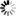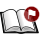Normal view

# A study on the electric dipole moment of atoms a thesis submitted for the degree of Doctor of Philosophy in the faculty of science Bangalore University Angom Dilip Kumar Singh [Ph.D Thesis]

Material type:TextPublication details: Bangalore Indian Institute of Astrophysics 1998Description: xii, 134pSubject(s): Online resources: Dissertation note: Doctor of Philosophy Bangalore University , Bangalore 1998 Summary: The forms of symmetries exhibited by physical systems can be classified as continuous and discrete. With each symmetry there is an associated transformation and these obey characteristic group structures. The continuous symmetries are associated with transformations which has the concept of infinitesimal transformation and a finite transformation can be achieved by applying a sequence of infinitesimal transformations. Whereas in discrete symmetries, there is no concept of infinitesimal transformations, instead the transformation takes the physical system from one state to another. The state of the system before and after the transformation can be same or different. If the system remains the same after the transformation then it is invariant under the particular symmetry, if the state is different after the transformation then the symmetry is said to be violated by the system[3}. In classical physics the discrete symmetries figure less prominently than they do in quantum physics . The three important discrete symmetries of interest in physical studies are charge conjugation(C), space-inversion/parity (P) and time-reversal(T). According to the CPT theorem[3} all physical systems described in local field theories are invariant under the combined CPT transformation. A physical system/process can violate each of these symmetries individually such that it is compensated by another of the symmetry but when combined the CPT-theorem is still valid. It has been now established that the weak interaction violates parity but to date no concrete evidence of T violation has been observed apart from the decay of kaons. Symmetry violations can be observed in laboratory by looking for their signatures in physical systems. For a single particle or a composite system the intrinsic Electric Dipole Moment(EDM) in a non-degenerate state is the signature of P and T violation. The significance of observing such an EDM is that it could lead to a better understanding of the origin of T or CP violation. Neutral Atomic systems are good candidates to search for EDM as it is a composite system of leptons and hadrons. The collective many-body effects can enhance the EDM arising from interaction in a certain sector or due to intrinsic EDM of the constituent particles either constructively or destructively. The effect of a particular interaction (property ) can be studied by an appropriate choice of atomic system. The enhancement factor is proportional to the atomic number Z and hence heavier atoms are preferred. Once an atom is chosen based on the physical effect of interest, the intrinsic EDM can be measured by subjecting the atom to a constant external electric field E. The interaction Hamiltonian between E and Da is -Da ·E. Experimentally Da is measured as the linear response to a constant external electric field. Though all the theoretical descriptions are applicable to both atomic systems as well as ionic systems, ions cannot be used in experiments as the external field gives rise to a net force acting on it. From now on only atomic systems will be considered. The atomic Hamiltonian is the sum of kinetic energy part of the electrons, electronnucleus coulomb interaction, .electron-electron coulomb interaction and the PT-violating interaction Hamiltonian HpTV ' The presence of HpTV implies that the eigen states of the atomic Hamiltonian are no more eigen-states of parity operator P. The theoretical computation is done by treating HpTV as the perturbation which mixes opposite parity eigen states of the unperturbed Hamiltonian Hatom , which has the kinetic energy of the electrons, electron-electron coulomb interaction and electron-nucleus coulomb interaction. This gives a mixed parity ground state of the atom, from which the intrinsic atomic EDM Da is computed as linear response to the external field.
Tags from this library: No tags from this library for this title.
Star ratingsAverage rating: 0.0 (0 votes)
Holdings
Item type Current library Shelving location Call number Status Date due BarcodeThesis & Dissertations IIA Library-Bangalore General Stacks 043.52/ SIN (Browse shelf(Opens below)) Available 15461

Thesis Supervisor B. P. Das

Doctor of Philosophy Bangalore University , Bangalore 1998

The forms of symmetries exhibited by physical systems can be classified as continuous and
discrete. With each symmetry there is an associated transformation and these obey characteristic group structures. The continuous symmetries are associated with transformations which has the concept of infinitesimal transformation and a finite transformation can be achieved by applying a sequence of infinitesimal transformations. Whereas in discrete symmetries, there is no concept of infinitesimal transformations, instead the transformation takes the physical system from one state to another. The state of the system before and after the transformation can be same or different. If the system remains the same after
the transformation then it is invariant under the particular symmetry, if the state is different after the transformation then the symmetry is said to be violated by the system[3}. In classical physics the discrete symmetries figure less prominently than they do in quantum physics . The three important discrete symmetries of interest in physical studies are charge
conjugation(C), space-inversion/parity (P) and time-reversal(T). According to the CPT
theorem[3} all physical systems described in local field theories are invariant under the
combined CPT transformation. A physical system/process can violate each of these symmetries individually such that it is compensated by another of the symmetry but when
combined the CPT-theorem is still valid. It has been now established that the weak interaction violates parity but to date no concrete evidence of T violation has been observed
apart from the decay of kaons. Symmetry violations can be observed in laboratory by
looking for their signatures in physical systems. For a single particle or a composite system
the intrinsic Electric Dipole Moment(EDM) in a non-degenerate state is the signature of
P and T violation. The significance of observing such an EDM is that it could lead to a
better understanding of the origin of T or CP violation.
Neutral Atomic systems are good candidates to search for EDM as it is a composite system of leptons and hadrons. The collective many-body effects can enhance the EDM arising
from interaction in a certain sector or due to intrinsic EDM of the constituent particles
either constructively or destructively. The effect of a particular interaction (property ) can
be studied by an appropriate choice of atomic system. The enhancement factor is proportional to the atomic number Z and hence heavier atoms are preferred. Once an atom
is chosen based on the physical effect of interest, the intrinsic EDM can be measured by
subjecting the atom to a constant external electric field E. The interaction Hamiltonian
between E and Da is -Da ·E. Experimentally Da is measured as the linear response to
a constant external electric field. Though all the theoretical descriptions are applicable to
both atomic systems as well as ionic systems, ions cannot be used in experiments as the
external field gives rise to a net force acting on it. From now on only atomic systems will
be considered.
The atomic Hamiltonian is the sum of kinetic energy part of the electrons, electronnucleus coulomb interaction, .electron-electron coulomb interaction and the PT-violating
interaction Hamiltonian HpTV ' The presence of HpTV implies that the eigen states of
the atomic Hamiltonian are no more eigen-states of parity operator P. The theoretical
computation is done by treating HpTV as the perturbation which mixes opposite parity
eigen states of the unperturbed Hamiltonian Hatom , which has the kinetic energy of the
electrons, electron-electron coulomb interaction and electron-nucleus coulomb interaction.
This gives a mixed parity ground state of the atom, from which the intrinsic atomic EDM
Da is computed as linear response to the external field.

There are no comments on this title.